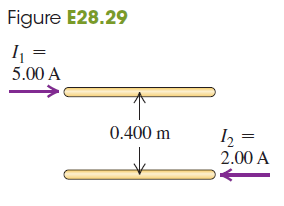# Problem: Two long, parallel wires are separated by a distance of 0.400 m (fig. E28.29). The currents I1 and I2 have the directions shown.(a) Calculate the magnitude of the force exerted by each wire on a 1.20-m length of the other. I s the for attractive or repulsive?(b) Each current is doubled, so that I1 becomes 10.0 A and I2 becomes 4.00 A. Now what is the magnitude of the force that each wire exerts on a 1.20-m length of the other?

###### FREE Expert Solution

The magnitude of the force per unit length between two-parallel current-carrying conductors is:

$\overline{)\frac{\mathbf{F}}{\mathbf{l}}{\mathbf{=}}\frac{{\mathbf{\mu }}_{\mathbf{0}}}{\mathbf{2}\mathbf{\pi }}\frac{{\mathbf{I}}_{\mathbf{1}}{\mathbf{I}}_{\mathbf{2}}}{\mathbf{d}}}$

Force, F:

$\overline{){\mathbf{F}}{\mathbf{=}}\frac{{\mathbf{\mu }}_{\mathbf{0}}}{\mathbf{2}\mathbf{\pi }}\frac{{\mathbf{I}}_{\mathbf{1}}{\mathbf{I}}_{\mathbf{2}}\mathbf{l}}{\mathbf{d}}}$

Since the current are in opposite direction, they will repel each other.

89% (320 ratings)###### Problem Details

Two long, parallel wires are separated by a distance of 0.400 m (fig. E28.29). The currents I1 and I2 have the directions shown.

(a) Calculate the magnitude of the force exerted by each wire on a 1.20-m length of the other. I s the for attractive or repulsive?

(b) Each current is doubled, so that I1 becomes 10.0 A and I2 becomes 4.00 A. Now what is the magnitude of the force that each wire exerts on a 1.20-m length of the other?